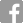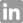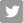×

# Prepare for the Quantitative Section

## Quantitative Reasoning Question Types, Strategies, and Resources

The Quantitative section measures your ability to analyze data and draw conclusions using reasoning skills. The mathematics needed to understand and solve the questions in this section of the GMAT exam is no greater than what is generally taught in secondary school classes. You will have 62 minutes to complete 31 multiple-choice questions.  The Quantitative section has two question types: Problem Solving and Data Sufficiency.

Your best performance starts with practicing with real GMAT questions from past exams using your FREE GMAT Official Starter Kit + Practice Exams 1 & 2. The Starter Kit includes tips and strategies for the Quantitative section as well as 30 Quantitative practice questions. You'll also have free access to two full-length, computer adaptive practice exams that use the same scoring algorithm as the real exam.

Review the Guide to GMAT Exam Prep Materials to find even more tools to help you prepare.

## What Math Skills Will You Need?

Doing well in the Quantitative Reasoning section of the GMAT exam involves knowing how to apply your knowledge of math to reasoning questions, including your knowledge of the following areas:

1. Arithmetic – basic areas of arithmetic, including integers, fractions, powers and roots, statistics, and probability
2. Algebra – topics, such as variables and functions, and how to solve different types of equations.
3. Geometry – the properties of geometric objects, including quadrilaterals, triangles, circles, solids, and cylinders, and coordinate geometry
4. Word problems – blending arithmetic, algebraic, and geometric principles to solve problems

## Problem Solving Question Strategies

Problem solving questions measure your ability to use logic and analytical reasoning to solve quantitative problems. You will solve the problem and indicate the best of five answer choices.

• Pace Yourself. Consult the on-screen timer periodically. Work as carefully as possible, but don’t spend valuable time checking answers or pondering problems you find difficult. It’s important to try to finish the section.
• Use the erasable noteboard provided at the test center to work out answers. Solving problems in writing may help you avoid errors.
• Read each question carefully to determine what data is given and what is being asked. For word problems, take one step at a time. Read each sentence carefully and translate the data into equations or other useful mathematical representations.
• Skim the answer choices before you answer a question. If you don’t, you may waste time putting answers in a form that’s not given.
• For questions that require approximations, skim the answer choices first. If you don’t get some idea of how close the approximation should be, you may waste time on long computations when a short mental process would serve you better.
• Don’t waste time by trying to solve a problem you recognize as too difficult or time-consuming. Eliminate the choices you know are wrong, select the best of the remaining choices, and move on to the next question.

## Data Sufficiency Question Strategies

Data Sufficiency questions measure your ability to analyze a quantitative problem, recognize which data are relevant, and determine at what point there is enough data to solve a problem.

• Decide whether the problem allows only one value or a range of values. Remember that you are only determining whether you have enough data.
• Avoid making unwarranted assumptions based on geometric figures. Figures are not necessarily drawn to scale.

### MBA Students Share Their Strategies in Preparing for the Quantitative Section of the GMAT™ Exam

The GMAT exam helps you stand out during the admissions process. Take the one business school exam that elevates you from the rest of the pack.

If test centers are closed in your area due to the COVID-19 pandemic, consider taking the GMAT Online Exam, an interim, proctored exam that you can take in the comfort of your home.

## Share;